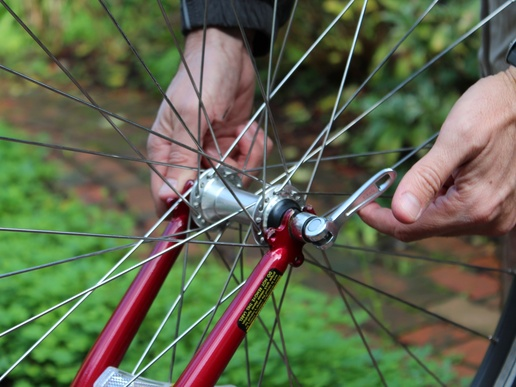Your browser doesn't support the features required by impress.js, so you are presented with a simplified version of this presentation.

For the best experience please use the latest Chrome, Safari or Firefox browser.

# Fish-based Composition

GitHub: possiblywrong

Bitbucket: dconlon

>=>
<=<
=>=
=<=
Recap on composition

`squggle ∘ bizzle === x => squggle( bizzle(x) )`

∘ : (B => C) => (A => B) => (A => C)
Why composition?

```val x = "someval"
for {
a <- someeffect(x)
b <- anothereffect(a)
c <- yetanothereffect(b)
} yield c
```
`val effects = yetanothereffect ∘ anothereffect ∘ someeffect`

Coupling != CompositionThink quick-release gears
```val x = "someval"
for {
a <- someeffect(x)
b <- anothereffect(a)
c <- yetanothereffect(b)
} yield c
```
```   someeffect(x).flatMap( anothereffect ).flatMap( yetanothereffect )
```

NB: flatMap takes an M[A] and a function A => M[B], and returns an M[B]

```   someeffect(x) >>= ( anothereffect ) >>= ( yetanothereffect )
```
```   val e = x => someeffect(x) >>= ( anothereffect ) >>= ( yetanothereffect )
```
```   e === Kleisli(someeffect) >==> anothereffect >==> yetanothereffect
```

NB: >==> != >=>, but lifts the RHS into kleisli

```   foo <=< bar === x => bar(x) >>= foo
```

A => M[B]

What about composing, M[A] => B ?

Use a spacebar or arrow keys to navigate